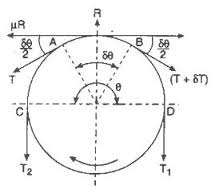# A classical mechanics problem by Tanay KibeConsider a pulley as shown. A string is placed on the pulley. The string is massless and the pulley has friction. Consider the two tensions $T_1$ and $T_2$, with $T_1>T_2$.

The coefficient of friction is $k$. Find the ratio $\dfrac{ T_1}{T_2}$ when the string is just about to slip over the pulley.

Details and Assumptions:

• Take $k =\frac1\pi , \theta = \pi$.

• Use the approximation $e = 2.71$.

×